# KSEEB SSLC Class 10 Maths Solutions Chapter 10 Quadratic Equations Ex 10.4

In this chapter, we provide KSEEB SSLC Class 10 Maths Solutions Solutions Chapter 10 Quadratic Equations Ex 10.4 for English medium students, Which will very helpful for every student in their exams. Students can download the latest KSEEB SSLC Class 10 Maths Solutions Solutions Chapter 10 Quadratic Equations Ex 10.4 pdf, free KSEEB SSLC Class 10 Maths Solutions Solutions Chapter 10 Quadratic Equations Ex 10.4 pdf download. Now you will get step by step solution to each question.

### Karnataka State Syllabus Class 10 Maths SolutionsChapter 10 Quadratic Equations Ex 10.4

Question 1.
Find the nature of the roots of the following quadratic equations. If the real roots exist, find them :
(i) 2x2 – 3x + 5 = 0
(ii) 3x2 – 43–√x + 4 = 0
(iii) 2x2 – 6x + 3 = 0
Solution:
i) 2x2 – 3x + 5 = 0
It is in the form of ax2 + bx + c = 0
a = 2, b = – 3 and c = 5
Nature of roots = b2 – 4ac
Discriminant = (- 3)2 – 4 × (2)(5)
= 9 – 40
= – 31
Discriminant = – 31 < 0
The given Quadratic equation has no real roots.

(ii) 3x2 – 43–√x + 4 = 0
Here, a = 3, b = 43–√, c = 4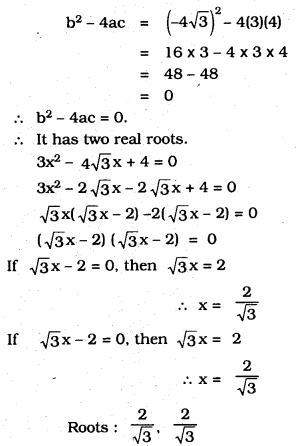(iii) 2x2 – 6x + 3 = 0
Here, a = 2, b = -6, c = 3
b2 – 4ac = (-6)2 – 4(2)(3)
= 36 – 24 = 12
∴ b2 – 4ac = 12 > 0.
∴ It has two distinct roots.
2x2 – 6x + 3 = 0
Here, a = 2, b = -3, c = 3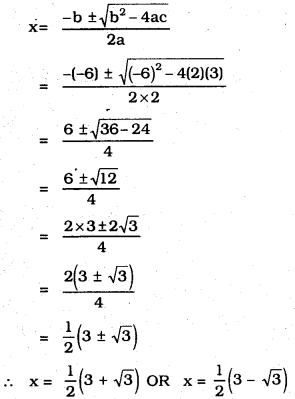Question 2.
Find the values of k for each of the following quadratic equations, so that they have two equal roots
(i) 2x2 + kx+3 = 0
(ii) kx(x – 2) + 6 = 0
Solution:
(i) Comparing the given quadratic equation with ax2+ bx + c = 0, we get a = 2,b = k,c = 3
∴ b2 – 4ac = (k)2 – 4(2)(3) = k2 – 24
∵ For a quadratic equation to have equal roots, b2 – 4ac = 0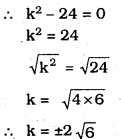(ii) kx(x – 2) + 6 = 0 ⇒ kx2 – 2kx + 6 = 0
Comparing kx2 – 2kx + 6 = 0 with ax2 + bx + c = 0, we get
a = k, b = -2k, c = 6
∴  b2 – 4ac = (-2k)2 – 4(k)(6) = 4k2 – 24k
Since, the roots are equal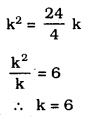But k cannot be 0, otherwise, the given equation is not quadratic. Thus, the required value of k is 6.

Question 3.
Is it possible to design a rectangular mango grove whose length is twice its breadth, and the area is 800 m2 ? If so, find its length and breadth.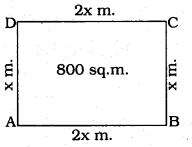Solution:
Let the breadth of rectangular mango grove is ‘x’ and length of rectangular mango grove is 2x
Area of rectangular mango grove = 800 m2
x (2x)= 800
2x2 = 800
x2 = 8002 = 400
x = ± 400−−−√
x = ± 20m
∴ breadth of mango grove x = 20 m
length of mango grove = 2x = 2 × 20 = 40 m
It is possible to construct a rectangular mango grove of length 40m and breadth 20m.

Question 4.
Is the following situation possible? If so, determine their present ages. The sum of the ages of two friends is 20 years. Four years ago, the product of their ages in years was 48.
Solution:
Let the age of one friend = x years
∴ Age of other friend = (20 – x) years
Four years ago,
Age of one friend = (x – 4) years
Age of other friend = (20 – x – 4) years
= (16 -x) years
According to the condition,
(x – 4) × (16 – x) = 48
⇒ 16x – 64 – x2 + 4x = 48
⇒ – x2 + 20x – 64 – 48 = 0
⇒ -x2 + 20x – 112 = 0
⇒ x2 – 20x + 112 = 0 …(1)
Comparing equation (1) with ax2 + bx + c = 0, we get a = 1, b = – 20 and c = 112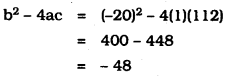Since, b2 – 4ac is less than 0.
∴ The quadratic equation (1) has no real roots.
Thus, the given situation is not possible.

Question 5.
Is it possible to design a rectangular park of perimeter 80 m. and area 400 m2? If so, find its length and breadth.
Solution: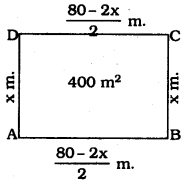Let the length of rectangular park is ‘x’
Perimeter of the rectangular park = 80m
2 (length + breadth) = 80
length + breadth = 802 = 40
area of rectangle = l × b
x (40 – x) = 400
40x – x2 = 400
x2 – 40x + 400 = 0
x2 – 20x – 20x + 400 = 0
x (x – 20) – 20 (x – 20) = 0
(x – 20) (x – 20) = 0
x – 20 (or) x – 20 = 0
x = 20 m
length of rectangular park = 20 m and breadth of rectangular park = (40 – x)
= 40 – 20 = 20m
∴ It is possible to design rectangular park of length 20 m and breadth is 20 m.
∴ The park is a square having 20m side.

All Chapter KSEEB Solutions For Class 10 Maths

—————————————————————————–

All Subject KSEEB Solutions For Class 10

*************************************************

I think you got complete solutions for this chapter. If You have any queries regarding this chapter, please comment on the below section our subject teacher will answer you. We tried our best to give complete solutions so you got good marks in your exam.

If these solutions have helped you, you can also share kseebsolutionsfor.com to your friends.

Best of Luck!!